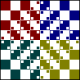## Function composition and \$ operator in Haskell

Today I was trying to understand how function application, composition and \$ work in Haskell. Consider two following lines of code:

```take 3 (reverse (filter even [1..10])) take 3 . reverse . filter even \$ [1..10]```

These are equivalent and both produce a list [10,8,6]. First version is based on grouping function calls with parentheses and this is pretty straightforward. My problem was the second version which is based on function composition and a special function application operator, denoted as `.` (dot) and `\$` respectively. This form is often used to write functions in a pointfree style1. I was trying to work out in what order function calls are executed and why do I have to use \$ operator. Here’s what I came up with.

The function composition (dot) operator is defined as:

```(.) :: (b -> c) -> (a -> b) -> a -> c (f . g) x = f (g x)```

This operator has priority of 9 and is right-associative2. This means that `a . b . c . d` is the same as `(a . (b . (c . d)))`. The `\$` operator is defined as:

```(\$) :: (a -> b) -> a -> b f \$ x = f x```

This operator simply applies a function to a given parameter. In contrast to standard function application, which has highest possible priority of 10 and is left-associative, the \$ operator has priority of 0 and is right-associative (that second property doesn’t matter in my example). Such a low priority means that all other operators on both sides of \$ will be evaluated before applying the \$. So the call

`take 3 . reverse . filter even \$ [1..10]`

will first evaluate `take 3 . reverse . filter even` constructing a partially applied function that becomes fully applied when it receives one more parameter. The missing parameter is the list on the right side of \$. By definition of dot operator, this call is therefore equivalent to `take 3 (reverse (filter even [1..10]))`. That’s what we expected.

Why do we need the \$ operator? If it wasn’t there then the function call `filter even [1..10]` would evaluate first – remember that function application has priority of 10, while function composition has a lower priority of 9. This would lead to `take 3 . reverse . [2,4,6,8,10]`, but a list cannot be composed with a function. The dot operator expects it’s second argument to be a function of one argument, not a list, and that’s the reason we need \$ – to allow function composition to evaluate first.

1. The name “pointfree” doesn’t come from the point operator used to compose functions []
2. You can verify this by typing `:i (.)` in ghci. This will display the type definition, fixity (infixr in that case) and priority of the dot operator []

### 8 Responses to “Function composition and \$ operator in Haskell”

1.Magnap says:

Thank you for this. I was reading “learn you a haskell for great good” and was wondering why you couldn’t just write take 3 \$ reverse \$ filter even \$ [1..10]

2.Jan Stolarek says:

Well, actually you can write that. “take 3 \$ reverse \$ filter even \$ [1..10]” is a valid Haskell code.

3.Rick Bradford says:

I had trouble understanding function composition until I redefined it in terms of pipelining.

Let’s write it :: [1..10] |> filter even |> reverse |> take 3

I find that more readable, but it is equivalent to:

take 3 . reverse . filter even \$ [1..10]

And, although Prelude doesn’t have pipelining built in, it can simply be defined:

(|>) f g = g f

Works like a charm, and makes my Haskell code much more readable

4.Matt says:

How does Haskell know to pass the function and parameter to \$ rather than pass \$ as a parameter to the function?

5.Jan Stolarek says:

To pass an operator as an argument to a function you have to put in parentheses, so that it becomes operator section with no arguments. So having:

`foo \$ bar`

Will pass `bar` as an argument to `foo`, whereas:

`foo (\$) bar`

will pass `(\$)` and `bar` as arguments to `foo`.

6.Fahad Aijaz says:

“Thank you for this. I was reading ?learn you a haskell for great good? and was wondering why you couldn?t just write take 3 \$ reverse \$ filter even \$ [1..10]”.

I would have to say exactly the same. Thanks

7.Tom Ellis says:

It’s not really about “evaluating”, just type checking.

8.Jarek Miszczak says:

Thanks for this post, very nice example with \$.

Staypressed theme by Themocracy